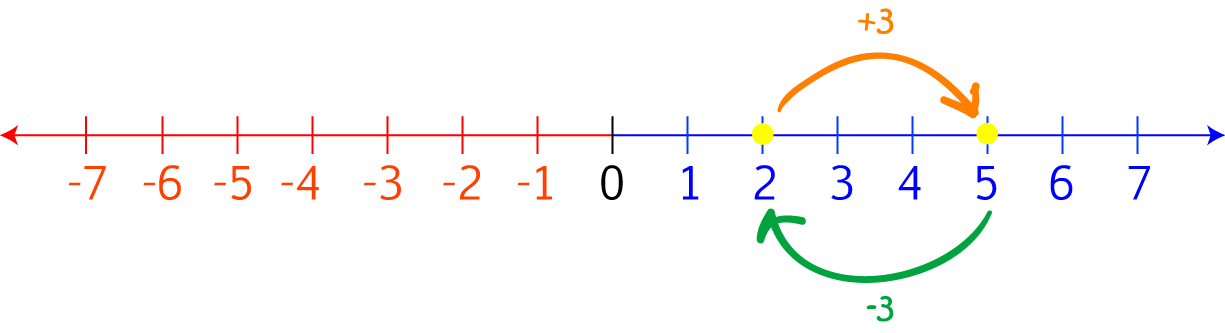# Definition of Inverse Operation

An inverse operation is an operation that undoes the work of another operation.

For example, addition and subtraction are inverse operations. If we start with $2$ and add $3$, then we end up at $5$. If we then subtract $3$, we end up back at $2$ again. So, subtracting $3$ has undone the work of adding $3$.

Multiplication (except by zero) and division are also inverse operations. For example, if we start with $3$ and multiply it by $2$, we end up with $6$. If we divide $6$ by $2$, we end up at $3$ again. So, dividing by $2$ has undone the work of multiplying by $2$.### Description

The aim of this dictionary is to provide definitions to common mathematical terms. Students learn a new math skill every week at school, sometimes just before they start a new skill, if they want to look at what a specific term means, this is where this dictionary will become handy and a go-to guide for a student.

### Audience

Year 1 to Year 12 students

### Learning Objectives

Learn common math terms starting with letter I

Author: Subject Coach
You must be logged in as Student to ask a Question.